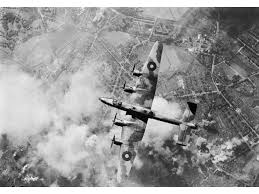# Queueing Theory: Bomber landing analysis

130520201343

The issue of queues was developed during World War II. It was about optimizing the landing process at English airports of great bubble squadrons returning from over the Third Reich. There were a lot of planes in relation to a limited number of airports. The formations had several hundred planes. Some of the planes were damaged or injured crew members were on board. Planes that are returning from combat usually had a small amount of fuel, which required immediate landing. This is how queue theory was born.

Queueing Theory is used to evaluate systems that should not be queued in theory. That is, systems in which the number of items per minute (λ) is less than the number of items handled per minute (μ), i.e. λ <μ.
If the number of inflowing elements is greater than the service speed: λ => μ then a queue appears.

In combat conditions, when the planes returned from the front there was no way there was a queue. The methodology described below is used to determine the likelihood that an aircraft will safely land in an environment where theoretically there is no queue.

The following formulas come from the study: Hillier F.S., Lieberman G.J. Instruction of Operation Research. New York: McGrew Hill 1990 oraz Z. Jędrzejczyk, K.Kukuła, J.Skrzypek, A. Walkosz Badania operacyjne w przykładach i zadaniach, Wydawnictwo Naukowe PWN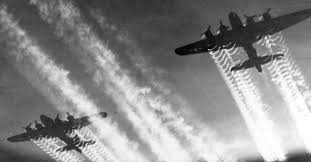# Arrival rate: λ

These are planes arriving within a minute.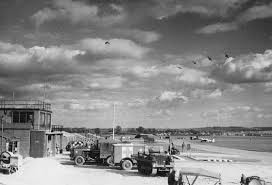In :
import numpy as np
import numpy as np

np.random.seed(148)
Λ = (np.random.random_sample(100)*1.0)

a= sum(Λ)
p= len(Λ)
λ = 1/(p/a)
print('Number of planes:     ', p)
print('Sume of landing time: %0.01f'% a)
print('Arrival_λ: %0.2f planes per min'% λ)
Λ[:14]

Number of planes:      100
Sume of landing time: 51.3
Arrival_λ: 0.51 planes per min

Out:
array([0.57540585, 0.14030841, 0.15827272, 0.06244513, 0.57575391,
0.60640513, 0.22672425, 0.91483505, 0.91555016, 0.89023892,
0.71296042, 0.56737137, 0.77315872, 0.02221362])

# Service rate: μ

In our example, this is the number of aircraft arrived in one minute.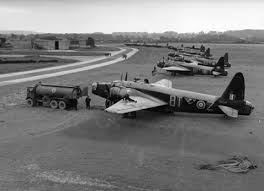In :
np.random.seed(148)
M = (np.random.random_sample(100)*1.2)

a= sum(M)
p= len(M)
μ = 1/(p/a)
print('Number of planes:     ', p)
print('Sume of service time: %0.01f min'% a)
print('Service_μ: %0.2f planes per min'% μ)
Λ[:14]

Number of planes:      100
Sume of service time: 61.6 min
Service_μ: 0.62 planes per min

Out:
array([0.57540585, 0.14030841, 0.15827272, 0.06244513, 0.57575391,
0.60640513, 0.22672425, 0.91483505, 0.91555016, 0.89023892,
0.71296042, 0.56737137, 0.77315872, 0.02221362])

# Traffic intensity: ϱ

Traffic intensity is the ratio of arrival rate to service rate.

$$\text{Traffic intensity equation:} \\ \bbox[#f3f3f3,12px,border:1px solid black] { {ϱ}=\frac{λ}{μ} } \qquad (1)$$
In :
ϱ = λ/μ
ϱ

if ϱ > 1:
print("The system is unstable, we do not carry out further calculations!")
print('Arrival rate λ > service rate μ')

else:
print("The system is stable, you can calculate the probabilities!")
print('Arrival rate λ > service rate μ')
print('Arrival rate λ:      %0.2f plane per one minut'%λ)
print('Service rate μ:      %0.2f plane per one minut'%μ)
print('Traffic intensity ϱ: %0.2f'%ϱ)


The system is stable, you can calculate the probabilities!
Arrival rate λ > service rate μ
Arrival rate λ:      0.51 plane per one minut
Service rate μ:      0.62 plane per one minut
Traffic intensity ϱ: 0.83


### The average number of airplanes waiting in the air for landing ψ:

$$\text{Average number of pending items:} \\ \bbox[#f3f3f3,12px,border:1px solid black] { ψ = \frac{?^2}{1-{?}} } \qquad (2)$$
In :
if ϱ > 1:
print("The system is unstable, we do not carry out further calculations!")
print('Arrival rate λ > service rate μ')
else:
ψ = ?**2/abs(1-?)
print('Average number of aircraft waiting≈ %0.2f '%ψ)

Average number of aircraft waiting≈ 4.17


### Probability of n pending items

The probability that there will be more than n waiting aircraft in the air.

$$\text{Probability of n pending items:} \\ \bbox[#f3f3f3,12px,border:1px solid black] { p(n>n_0)= {?}^{{n_0}+1} } \qquad (3)$$

What is the probability that there are more than 3 planes in the air?

In :
n=3

In :
if ϱ > 1:
print("The system is unstable, we do not carry out further calculations!")
print('Arrival rate λ > service rate μ')
else:
p3 = ?**(n+1)
print('The probability that there are more than 3 planes in the air≈ %0.2f '%p3)

The probability that there are more than 3 planes in the air≈ 0.48


### Probability of longer waiting than t¶

The time the aircraft waits for permission to land.

$$\text{Probability of longer waiting than t:} \\ \bbox[#f3f3f3,12px,border:1px solid black] { p(t>t_0)= {? e}^{-t_0 (μ-λ)} } \qquad (4)$$

What is the probability that the plane will wait for a landing over 3 minut?

In :
## time in minutes
t=3

In :
if ϱ > 1:
print("The system is unstable, we do not carry out further calculations!")
print('Arrival rate λ > service rate μ')
else:
p4 = ?*np.e**(-t*(μ-λ))
print('The probability that there are more than 3 planes in the air ≈ %0.2f '%p4)

The probability that there are more than 3 planes in the air ≈ 0.61


# Multiple service channel¶

We assume in this algorithm that the arrival of aircraft (arrival rate) has a normal distribution. Planes are directed to several nearby airports with the same level of service. The planes form a joint queue and fly to this airport, where a place has just vacated. To maintain system balance, inequality must be maintained:

\begin{align} λ < rμ &&\text{where r is the number of service channels} \tag 5\\ \end{align}

$$\text{The probability that there are no queues at r service stations:} \\ \bbox[#f3f3f3,12px,border:1px solid black] { p(n=0) = \displaystyle\frac{1}{sum_{n=1}^\infty} } \qquad (6)$$

The probability that there are no queues at r service stations.

$$\text{The probability that there is no queue n = 0 in the system:} \\ \bbox[#f3f3f3,12px,border:1px solid black] { p(n=0) =\frac{1}{\displaystyle\sum_{i=0}^{r-1} \frac{?^i}{i!}+\frac{?^r}{{\left(r-{?}\right)}{\left(r-1\right)!}}} } \qquad (7)$$
$$\text{Average number of items waiting in queue:} \\ \bbox[#f3f3f3,12px,border:1px solid black] { ψ =\frac{{?^{r+1}{p(n=0)}}}{{(r-?)}^2{(r-1)!}} } \qquad (8)$$
$$\text{The probability that there is no queue n = 0 in the system:} \\ \bbox[#f3f3f3,12px,border:1px solid black] {p(n) =\begin{cases} \frac{{ψ^n}{p(n=0)}}{n!}, & \text{if n\le r} \\[2ex] \frac{{r^{r-n}}{ψ^n}{p(n=0)}}{r!}, & \text{if n\gt r} \\[2ex] \end{cases} } \qquad (9)$$

The probability that the system has more than zero elements and elements is more than r channels.

$$\text{The probability that there is no queue n = 0 in the system:} \\ \bbox[#f3f3f3,12px,border:1px solid black] { p(n>n_0) =\frac{{r^{r-n_{0}}}{?^{n_{0}+1}}{p(n=0)}}{(r-{?})r!} } \qquad (10)$$
$$\text{The probability that the waiting time of an item in the queue is longer n that t.:} \\ \bbox[#f3f3f3,12px,border:1px solid black] { p(t>t_0) ={{p{(n>r-1)}}{e^{μt(r-ψ)}}} } \qquad (11)$$

We check for inequality:

# λ<rμ¶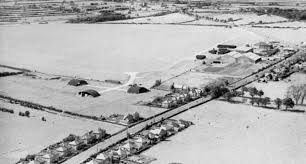In :
r=2
λ<r*μ

Out:
True
In :
print('Arrival rate λ:      %0.2f'%λ)
print('Service rate μ:      %0.2f'%μ)
ϱ = λ/(r*μ)
print('Traffic intensity ϱ: %0.2f'%ϱ)

Arrival rate λ:      0.51
Service rate μ:      0.62
Traffic intensity ϱ: 0.42

In :
if ϱ > 1:
print("The system is unstable, we do not carry out further calculations!")
print('Arrival rate λ > split service rate μ')
else:
print("The system is stable, you can calculate the probabilities!")
print('Arrival rate λ > split service rate μ')

The system is stable, you can calculate the probabilities!
Arrival rate λ > split service rate μ

In :
def factorial(n):
return 1 if (n==1 or n==0) else n * factorial(n - 1);


Since there are two airports, the time to accept the plane is 2*μ

### Probability of no queues, pattern no. 7¶

$${ p(n=0) =\frac{1}{\displaystyle\sum_{i=0}^{r-1} \frac{?^i}{i!}+\frac{?^r}{{\left(r-{?}\right)}{\left(r-1\right)!}}} }\qquad (7)$$

In :
if ϱ > 1:
print("The system is unstable, we do not carry out further calculations!")
print('Arrival rate λ > split service rate μ')
else:
p7=1/((1+ϱ)+(((ϱ**r)/((r-ϱ)*factorial(r-1)))))
print('Probability of no queues ?(?=0)≈ %0.2f'%p7)

Probability of no queues ?(?=0)≈ 0.66


### Probability that you will have to wait in queue, template no. 10¶

$${ p(n>n_0) =\frac{{r^{r-n_{0}}}{?^{n_{0}+1}}{p(n=0)}}{(r-{?})r!} } \qquad (10)$$

In :
if ϱ > 1:
print("The system is unstable, we do not carry out further calculations!")
print('Arrival rate λ > split service rate μ')
else:
n0 = 0
p10 = ((r**(r-n0))*(?**(n0+1))*p7)/((r-?)*factorial(r))
print('Probability to wait in queue ?(?>?0)≈ %0.2f'%p10)

Probability to wait in queue ?(?>?0)≈ 0.34


### Probability of two items in the queue: $p(n>2)$ where $n_0 =2$¶

In :
n0 = 2

In :
if ϱ > 1:
print("The system is unstable, we do not carry out further calculations!")
print('Arrival rate λ > split service rate μ')
else:
n0 = 2
p11 = ((r**(r-n0))*(?**(n0+1))*p7)/((r-?)*factorial(r))
print('Probability of two items in the queue: ?(?>2)≈ %0.2f'%p11)

Probability of two items in the queue: ?(?>2)≈ 0.01


### The probability that the item will have to queue over k minutes: $p(t>?_0)$¶

$${ p(n>n_0) =\frac{{r^{r-n_{0}}}{?^{n_{0}+1}}{p(n=0)}}{(r-{?})r!} } \qquad (10)$$

$${ p(t>t_0) ={{p{(n>r-1)}}{e^{μt(r-ψ)}}} } \qquad (11)$$

In :
## time in minutes
t0=10
t0=t0/60

print('n - unspecified number of units in the queue')
print('number of channels r =', r)

n - unspecified number of units in the queue
number of channels r = 2


$p(n>r-1) = p(n>1)$
equation ⟹ 10 for $p(n>1)$

In :
n0=1
p12 = ((r**(r-n0))*(?**(n0+1))*p7)/((r-?)*factorial(r))
p12

Out:
0.0718390804597701
In :
# wzór 11
if ϱ > 1:
print("The system is unstable, we do not carry out further calculations!")
print('Arrival rate λ > split service rate μ')
else:
p13 = p12*(np.e**(-μ*t0*(r-?)))
print('The probability that the item will have to queue over n minutes: ?(?>?0)≈ %0.2f'%p13)

The probability that the item will have to queue over n minutes: ?(?>?0)≈ 0.06


### What is the average number of plane waiting?!¶

${ ψ =\frac{{?^{r+1}{p(n=0)}}}{{(r-?)}^2{(r-1)!}} } \qquad (8)$

In :
if ϱ > 1:
print("The system is unstable, we do not carry out further calculations!")
print('Arrival rate λ > split service rate μ')
else:
p14 = ((?**(r+1))*p7)/(((r-?)**2)*factorial(r-1))
print('The average number of items waiting ψ ≈ %0.2f'%p14)

The average number of items waiting ψ ≈ 0.02